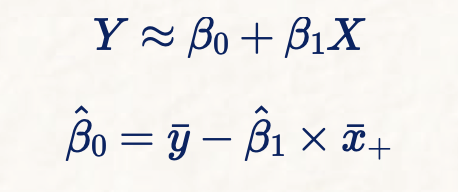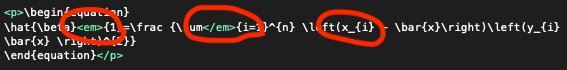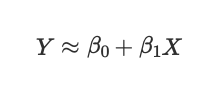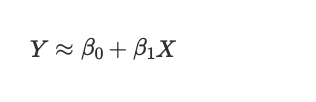# Unable to render some Latex markup

How can I troubleshoot not being able to render Latex in the MD file?

I have this equation, that renders just fine in the markdown file in the DT AND on Overleaf:

\begin{equation}
Y \approx \beta_{0}+\beta_{1} X
\end{equation}

and then in the very same document I have this equation:

\begin{equation}
\hat{\beta}_{0} = \bar{y} - \hat{\beta}_{1} \times \bar{x}_{+}
\end{equation}


that renders just fine on Overleaf but would NOT render in DT.
Is there some syntax that DT does not support?

I copied/pasted your second set into a new MD file in DEVONthink 3.8 with MathJax enabled. When I removed the bold asterisks (**) on the begin and end text …

\\begin\{equation\} Y \\approx \\beta\_\{0\}+\\beta\_\{1\} X \\end\{equation\}

\\begin\{equation\}
\\hat\{\\beta\}\_\{0\} = \\bar\{y\} - \\hat\{\\beta\}\_\{1\} \\times \\bar\{x\}\_\{+\}
\\end\{equation\}


it rendered as shown here:Ok, this is really weird. I converted the MD file to HTML and then looked at the source code.

For a very strange reason DT converted first two underscores used to denote subscript to <em> tags, but left the other underscores intact. So I had to escape just these first two underscores to render the equation properly.<p>\begin{equation}
\hat{\beta}<em>{1}=\frac {\sum</em>{i=1}^{n} \left(x_{i} - \bar{x}\right)\left(y_{i} - \bar{y}\right)}{\sum_{i=1}^{n}\left(x_{i} - \bar{x} \right)^{2}}
\end{equation}</p>


change to this:

\begin{equation}
\hat{\beta}\_{1}=\frac {\sum\_{i=1}^{n} \left(x_{i} - \bar{x}\right)\left(y_{i} - \bar{y}\right)}{\sum_{i=1}^{n}\left(x_{i} - \bar{x} \right)^{2}}
\end{equation}


So although I solved the problem, I’m not really sure what to do next time I have such a problem. Should I escape all underscores? First two or three?

I’m not sure where the asterisks came from, there are definitely none in my markup.
Why do you use two slashes? Would not that escape the second slash?

I just did as I said: copy/pasted from your post into a new MD document, and for the offending LaTeX I removed the ** bold astericks that you (or something) posted). I didn’t add the extra backslashes–came in on the copy/paste–frankly, did not notice since it worked first try.

I did not do anything with HTML.

I’m at the end of my knowledge of this.

To clarify, they are included in what you posted and what I copy/pasted. And I did not escape underscores, nor notice the double backslashes. Just copy/paste less bold markdown code what you posted.

By default DEVONthink (now) automatically escapes RTF if pasted into Markdown.

There’s a hidden preference RawMarkdownPasting to disable the automatic conversion, see this post.

2 Likes

MathJax and Katex and the likes do not support everything and of course all the various LaTeX modules cannot be supported. Using Markdown applications with MathJax support and the likes you have to know which LaTeX delimiters are set or supported.

$Y \approx \beta_{0}+\beta_{1} X$Convert to HTML you should get

of course, the HTML loads MathJax which converts the LaTeXthe delimeters to start and stop LaTeX in Markdown tend to be

For inline: $...$ $$....$$ \$......\$
For block: $$.....$$ $.....$ \$.....\$ \begin{equation}.....\end{equation} \begin{align} .....\end{align}

As I said not all these work with different applications and developer libraries.
The best applications allow you to set the preferred.

1 Like

Yes, that’s pretty much what I figured.
I was just bewildered why just first two underscores were converted to <em> tags and not all.

BTW, do you happen to know how to force a new line in the equation?
It seems that MathJax does not support double slashes \\ to force a new line inside the equation block.

Got it - just had to escape double slashes: \\\\

I use markdown so i just use  \\